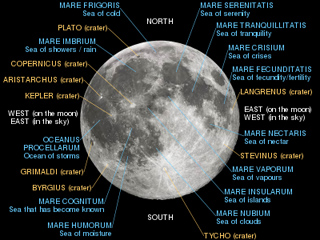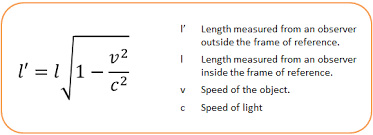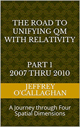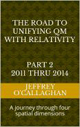# Einstein’s quantum probability function

I like to think the moon is there even if I am not looking at it is one of the more famous quotes attributed to Einstein when confronting the Quantum mechanical assumption that objects do not exist is space and time until they are observed.

Quantum mechanics assumes that one cannot define the position of particle in terms of where is has been but only in terms of the probabilistic values associated with its wave function.  This is in stark contrast to the Classical "Newtonian" assumption that one can assign precise values of future events based on the knowledge of their past.For example in a quantum system SchrÃ¶dinger wave equation plays the role of the classical Newtonian laws in that it predicts the future position or momentum of a particle in terms of a probability distribution by assuming that it simultaneously exists everywhere in three-dimensional space before it is observed.

This accentuates the fundamental difference between quantum and classical mechanics because the latter tells that a particle and the moon do not exist in specific position until observed where as Classical mechanics tell us that it does.

However, Einstein unknowing may have provided a way to define the classical "reality" of quantum probabilities by extrapolating the laws of a classical mechanics to the physical properties of the space-time environment he defined.

One of the reasons he may have been unaware of this possibility is because the probability function of quantum mechanics address the spatial properties of position whereas he chose to define the universe in terms of the time properties of four dimensional space-time.  In other words understanding the physical connection between the spatial properties of quantum mechanics and the time properties of Einstein space-time universe is extremely difficult for the same reasons as one would find it difficult to define a physical connection between apples and oranges.

Yet, he gave us the solution to this problem when he used the equation E=mc^2 and the constant velocity of light to define the geometric properties of particle in a space-time universe because that provided a method of converting a unit of time he associated with energy to unit of space one can associate with position in four *spatial* dimensions.  Additionally because the velocity of light is constant he also defined a one to one quantitative and qualitative correspondence between his space-time universe and one made up of four *spatial* dimensions.

However, as was just mentioned this change in perspective allows one to define a physical connection between Einstein theories and the probability functions of quantum mechanics in terms of their common spatial properties.

For example in the article “Why is energy/mass quantized?” Oct. 4, 2007 it was shown one can derive why the energy associated with the probability wave of quantum mechanics appears as a particle when observed by extrapolating the laws of classical wave mechanics in a three-dimensional environment to a energy wave on a "surface" of a three-dimensional space manifold with respect to  a fourth *spatial* dimension.

Briefly it showed the four conditions required for resonance to occur in a classical environment, an object, or substance with a natural frequency, a forcing function at the same frequency as the natural frequency, the lack of a damping frequency and the ability for the substance to oscillate spatial would occur in one consisting of four spatial dimensions.

The existence of four *spatial* dimensions would give an energy wave the ability to oscillate spatially on a "surface" between a third and fourth *spatial* dimensions thereby fulfilling one of the requirements for classical resonance to occur.

These oscillations would be caused by an event such as the decay of a subatomic particle or the shifting of an electron in an atomic orbital.  This would force the "surface" of a three-dimensional space manifold to oscillate with the frequency associated with the energy of that event.

The oscillations caused by such an event would serve as forcing function allowing a resonant system or "structure" to be established space.

Therefore, these oscillations in a "surface" of a three-dimensional space manifold would meet the requirements mentioned above for the formation of a resonant system or "structure" in four-dimensional space if one extrapolated them to that environment.

Classical mechanics tells us the energy of a resonant system can only take on the discrete or quantized values associated with its fundamental or a harmonic of its fundamental frequency.

Hence, these resonant systems in four *spatial* dimensions would be responsible for the discrete quantized energy associated with quantum mechanical systems.

Yet one can also define the boundary conditions responsible for a creating a particle in the terms of our perceptions of a three-dimensional environment.

For example in our three-dimensional world, a point on the two-dimensional surface of paper is confined to that surface. However, that surface can oscillate up or down with respect to three-dimensional space.

Similarly an object occupying a volume of three-dimensional space would be confined to it however, it could, similar to the surface of the paper oscillate “up” or “down” with respect to a fourth *spatial* dimension.

The confinement of the “upward” and “downward” oscillations of an electromagnetic wave with respect to a fourth *spatial* dimension is what defines the spatial boundaries associated with the resonant system the article "Why is energy/mass quantized?" Oct. 4, 2007 associates with a particle.

This give us explanation of why, in a quantum system the intervention of an observer forces it to "choose" a state or how it "knows" when someone is observing it because if a particle is free to move it will display its wave characteristics while in every case, observing it requires one to confine its energy to the specific volume associated with the observing equipment. Therefore it will always display its particle "reality" when someone looks or observes it.

However one of the most advantageous results of viewing the relativistic properties of Einstein’s theories in terms of their spatial instead of their time components is that it allow for the integration of one of most perplexing aspects of quantum mechanics; that of how and why a particle’s position when observed is based on probabilities and how it can exist simultaneously exists everywhere.

The physics of wave mechanics tell us, due to the continuous properties the energy waves the article "Why is energy/mass quantized?" Oct. 4, 2007 associated with a quantum system it would be distributed throughout the entire "surface" a three-dimensional space manifold with respect to a fourth *spatial* dimension.

For example the energy of a vibrating or oscillating ball on a rubber diaphragm would be disturbed over its entire surface while the magnitude of those vibrations would decrease as one move away from the focal point of the oscillations.

Similarly if the assumption that wave function of quantum mechanics represents vibrations or oscillations in a "surface" of three-dimensional space, as was mentioned earlier is correct these oscillations would be distributed over the entire "surface" three-dimensional space while the magnitude of those vibrations would be greatest at the focal point of the oscillations and decreases as one moves away from it.

As was also mentioned earlier the article “Why is energy/mass quantized?” showed a quantum particle is a result of a resonant structure formed on the "surface" of a three-dimensional space manifold with respect to a fourth *spatial* dimension.

Yet the science of Wave Mechanics tells us resonance would most probably occur on the surface of the rubber sheet were the magnitude of the vibrations is greatest and would diminish as one move away from that point,

Similarly the resonant structure that article associated with a particle would most probably be found were the magnitude of the vibrations in a "surface" of a three-dimensional space manifold is greatest and would diminish as one move away from that point.

In other words the position in space and time of a single particle could only be defined in terms of the probabilities associated with quantum mechanics.

Additionally Einstein theory also gives us the answer as to why a particle simultaneously exists everywhere in three-dimensional space.

That theory tell us all energy waves such as that the article "Why is energy/mass quantized?" Oct. 4, 2007 defined as being responsible for a particle travel at the speed of light.

However it also tells the length of an object relative to another is effected by its relative velocity and that that there is no preferred reference frames by which one can measure that length. Therefore one must not only view the distance traversed by the wave with respect to an observer who was external to it but one must also view distances from the wave’s perspective.Yet he also tells us that the length of everything including the universe from an object or wave moving at the speed of light is zero as can be seen from his formal on the right for length contraction.

Therefore from the perspective of the energy wave the article "Why is energy/mass quantized?" (mentioned earlier) was showed responsible for a particle, the distance or length between the end point of the entire universe is zero.

In other words from the perspective of the energy wave responsible for a particle the physical length of the universe is zero, therefore it exists at every point in it.  In other words Einstein theory tells it must simultaneously everywhere when observed by an observer who is not moving at the speed of light.  This gives us an explanation in terms of physical properties of Einstein’s space-time universe for the validity of quantum mechanics assumption that a particle simultaneously exists everywhere in three-dimensional space before being observed.  In other words it only "decides" where it wants to be in space when it is prevented from moving at the speed of light relative to an observer by an observation.

There can be no other interpretation if one accepts his Theory of Relativity.

Finally one can definitively answer Einstein’s question "Is the moon there even if I am not looking at it" in terms of his Theory of Relativity.

As was shown earlier a individual particle would most probably be found were the magnitude of the vibrations associated with it’s wave packet is the greatest.  Therefore the position of the mass components of all objects that consist of multiple particles such as the moon would be the point in space where the energy of their individual wave packets overlap which would result that point having a larger energy concentration than the sounding space.

However he did not define the location of a mass, such as the moon in terms of it’s quantized properties but in terms of how energy is concentrated at the apex of a curvature in the continuous properties space-time.

This tells us the moon is there when we are not looking because the overlapping of its individual energy wave components causes their energy to be concentrated in a specific volume of three-dimensional space and not because of the probability it’s individual particle components will be at that specific spot in space.

This shows that one can define why the quantum probability function gives us an accurate description of nature and why the moon is their when no is looking by extrapolating the laws of a classical mechanics to the physical properties of the space-time environment defined by Einstein.

Later Jeff

 The Road to Unifying QM with Relativity part 1 2007 thru 2010Ebook \$8.00Paper Back \$15.00 The Road to Unifying QM with Relativity part 2 2011 thru 2014Ebook \$8.00Paper Back \$16.00 The Road to Unifying QM with Relativity part 3 2015 thru 2020Ebook \$8.00Paper Back \$18.00